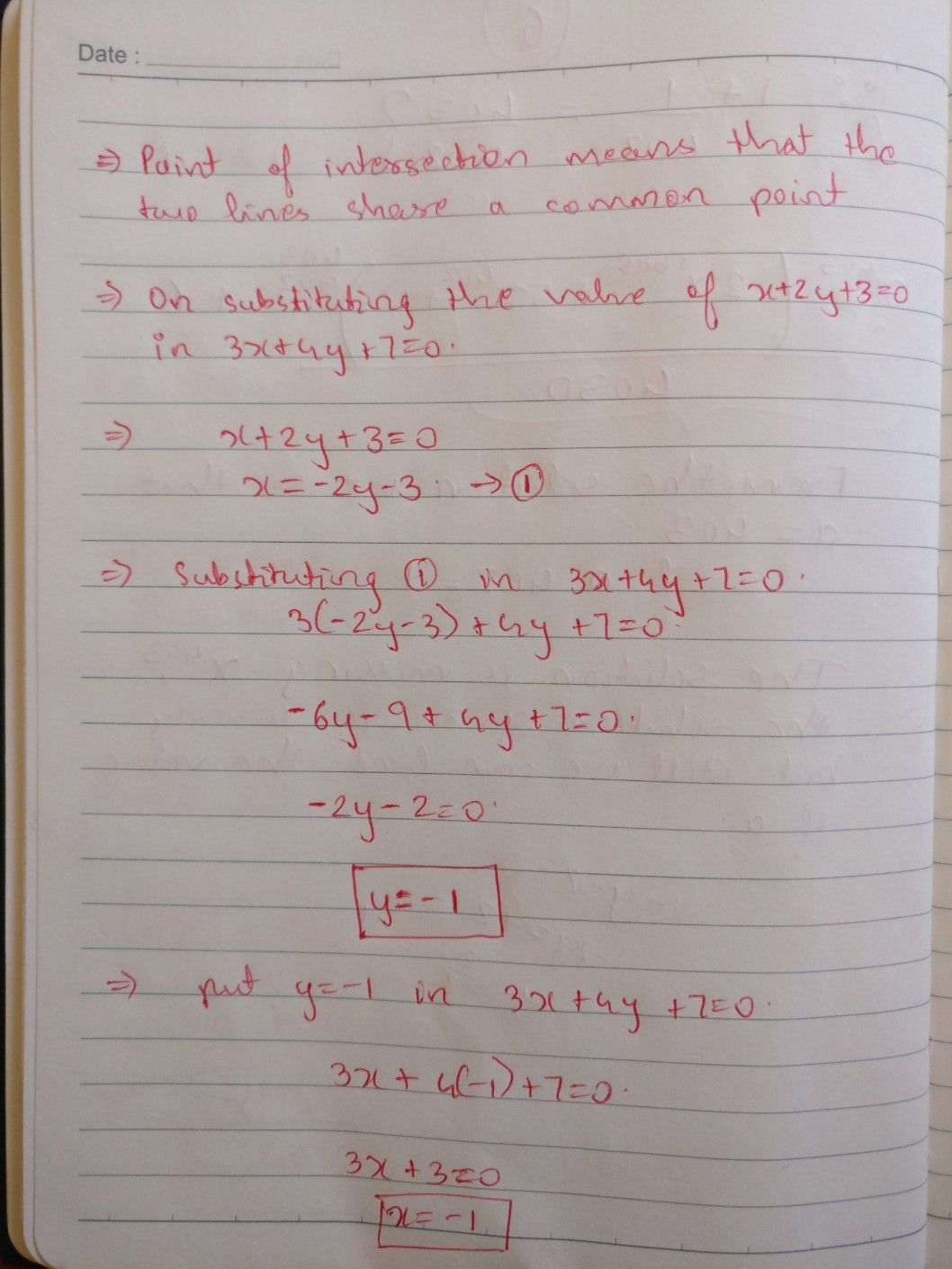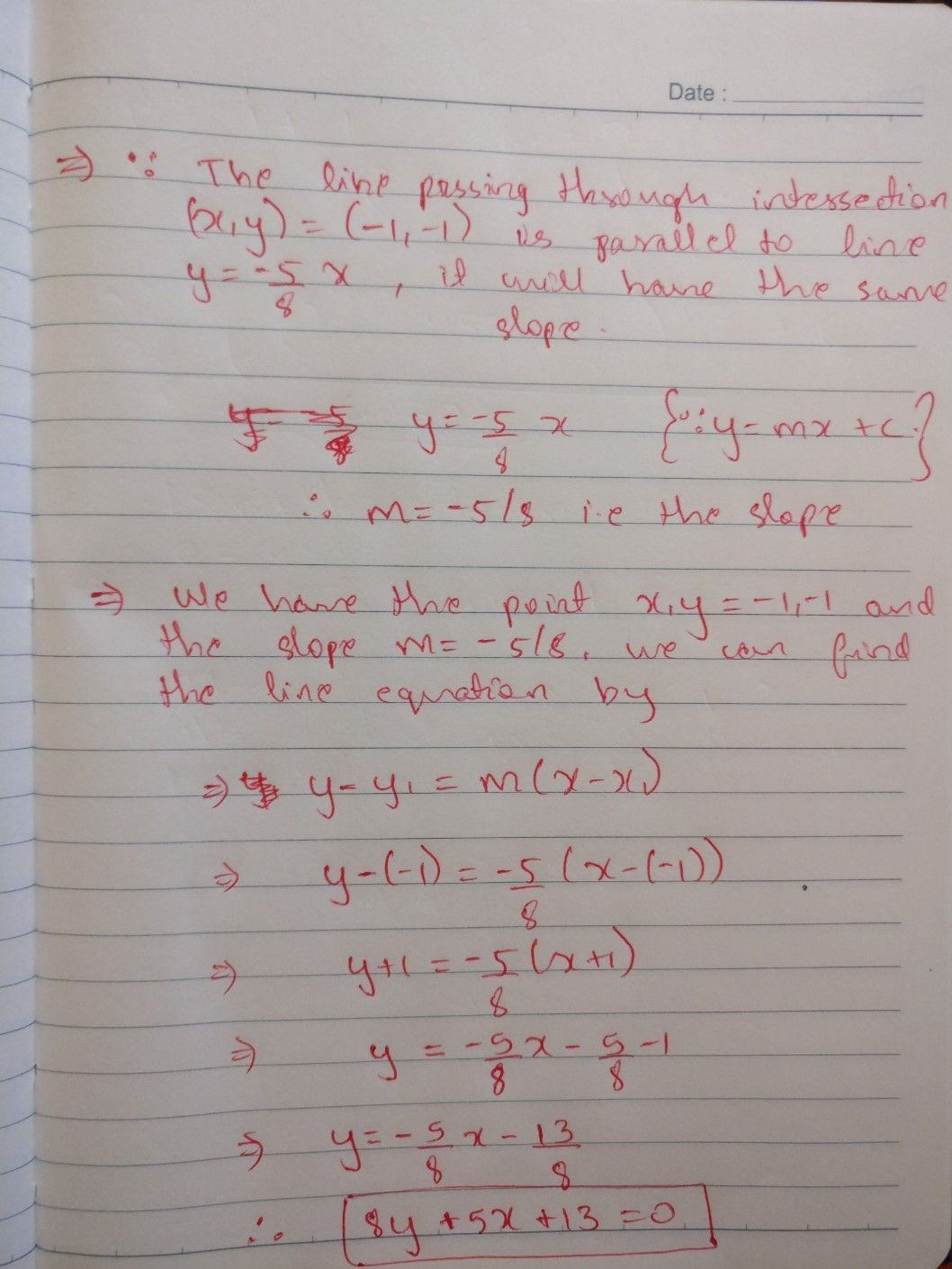Symbol
Problem$i$ . Find the equation of the straight line passing through the point of intersection of the straight lines $x+2y+3=0$ and $3x+4y+7=0$ and parallel to the straight line $y=-\dfrac {5} {8}x$ $a$